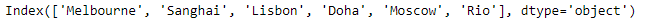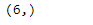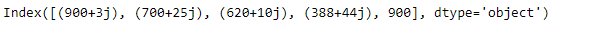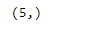# Python | Pandas Index.shape

• Last Updated : 20 Feb, 2019

Pandas Index is an immutable ndarray implementing an ordered, sliceable set. It is the basic object which stores the axis labels for all pandas objects.

Pandas` Index.shape` attribute return a tuple of the shape of the underlying data in the given Index object.

Syntax: Index.shape

Parameter : None

Returns : tuple

Example #1: Use `Index.shape` attribute to return a tuple of the shape of the underlying data in the given Index object.

 `# importing pandas as pd``import` `pandas as pd`` ` `# Creating the index``idx ``=` `pd.Index([``'Melbourne'``, ``'Sanghai'``, ``'Lisbon'``, ``'Doha'``, ``'Moscow'``, ``'Rio'``])`` ` `# Print the index``print``(idx)`

Output :Now we will use `Index.shape` attribute to return a tuple of the shape of the underlying data in the given Index object.

 `# return a tuple of the shape``# of data in idx object``result ``=` `idx.shape`` ` `# Print the result``print``(result)`

Output :As we can see in the output, the `Index.shape` attribute has returned a tuple based on the size of the underlying data in the given Index object.

Example #2 : Use `Index.shape` attribute to return a tuple of the shape of the underlying data in the given Index object.

 `# importing pandas as pd``import` `pandas as pd`` ` `# Creating the index``idx ``=` `pd.Index([``900` `+` `3j``, ``700` `+` `25j``, ``620` `+` `10j``, ``388` `+` `44j``, ``900``])`` ` `# Print the index``print``(idx)`

Output :Now we will use `Index.shape` attribute to return a tuple of the shape of the underlying data in the given Index object.

 `# return a tuple of the shape``# of data in idx object``result ``=` `idx.shape`` ` `# Print the result``print``(result)`

Output :As we can see in the output, the `Index.shape` attribute has returned a tuple based on the size of the underlying data in the given Index object.

My Personal Notes arrow_drop_up